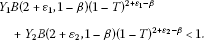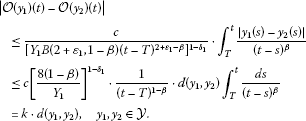Theory and Modern Applications

# On a fractional differential equation with infinitely many solutions

## Abstract

We present a set of restrictions on the fractional differential equation ${x}^{\left(\alpha \right)}\left(t\right)=g\left(x\left(t\right)\right)$, $t\ge 0$, where $\alpha \in \left(0,1\right)$ and $g\left(0\right)=0$, that leads to the existence of an infinity of solutions (a continuum of solutions) starting from $x\left(0\right)=0$. The operator ${x}^{\left(\alpha \right)}$ is the Caputo differential operator.

## 1 Introduction

The issue of multiplicity for solutions of an initial value problem that is associated to some nonlinear differential equation is essential in the modeling of complex phenomena.

Typically, when the nonlinearity of an equation is not of Lipschitz type , there are only a few techniques to help us decide whether an initial value problem has more than one solution. As an example, the equation ${x}^{\mathrm{\prime }}=f\left(x\right)=\sqrt{x}\cdot {\chi }_{\left(0,+\mathrm{\infty }\right)}\left(x\right)$ has an infinity of solutions (a continuum of solutions , p.15]) ${x}_{T}\left(t\right)=\frac{{\left(t-T\right)}^{2}}{4}\cdot {\chi }_{\left(T,+\mathrm{\infty }\right)}\left(t\right)$ defined on the nonnegative half-line which start from $x\left(0\right)=0$. Here, by χ we denote the characteristic function of a Lebesgue-measurable set.

An interesting classical result [1, 10], which generalizes the example, asserts that the initial value problem

$\left\{\begin{array}{cc}{x}^{\mathrm{\prime }}\left(t\right)=g\left(x\left(t\right)\right),\hfill & t\ge 0,\hfill \\ x\left(0\right)={x}_{0},\hfill & {x}_{0}\in \mathbb{R},\hfill \end{array}$
(1)

where the continuous function $g:\mathbb{R}\to \mathbb{R}$ has a zero at ${x}_{0}$ and is positive everywhere else, possesses an infinity of solutions if and only if ${\int }_{{x}_{0}+}\frac{du}{g\left(u\right)}<+\mathrm{\infty }$.

Recently, variants of this result have been employed in establishing various facts regarding some mathematical models [13, 14]. In particular, if the function g is allowed to have two zeros ${x}_{0}<{x}_{1}$ while remaining positive everywhere else and

${\int }_{{x}_{0}+}\frac{du}{g\left(u\right)}<+\mathrm{\infty },\phantom{\rule{2em}{0ex}}{\int }^{{x}_{1}-}\frac{du}{g\left(u\right)}=+\mathrm{\infty },$

then the problem (1) has an infinity of solutions ${\left({x}_{T}\right)}_{T>0}$ such that ${lim}_{t\to +\mathrm{\infty }}{x}_{T}\left(t\right)={x}_{1}$.

Our intention in the following is to discuss a particular case of the above non-uniqueness theorem in the framework of fractional differential equations. To the best of our knowledge, the result has not been established in its full generality yet for any generalized differential equation. We mention at this point the closely connected investigation .

In the last number of years, it became evident that differential equations of non-integer order, also called fractionals (FDE’s), can capture better in models many of the relevant features of complex phenomena from engineering, physics or chemistry, see the references in [24, 6, 7, 9, 11, 12, 16].

Let us consider a function $h\in {C}^{1}\left(I,\mathbb{R}\right)\cap C\left(\overline{I},\mathbb{R}\right)$ with ${lim}_{t↘0}\left[{t}^{1-\alpha }{h}^{\mathrm{\prime }}\left(t\right)\right]\in \mathbb{R}$ for some $\alpha \in \left(0,1\right)$, where $I=\left(0,1\right]$. The Caputo derivative of order α of h is defined as

${h}^{\left(\alpha \right)}\left(t\right)=\frac{1}{\mathrm{\Gamma }\left(1-\alpha \right)}\cdot {\int }_{0}^{t}\frac{{h}^{\mathrm{\prime }}\left(s\right)}{{\left(t-s\right)}^{\alpha }}\phantom{\rule{0.2em}{0ex}}ds,\phantom{\rule{1em}{0ex}}t\in I,$

where Γ is Euler’s function Gamma, cf. , p.79]. To have an idea about the eventual smoothness of this quantity, we mention that by letting the function ${h}^{\mathrm{\prime }}$ be at least absolutely continuous , Chapter 7] the (usual) derivative of ${h}^{\left(\alpha \right)}$ will exist almost everywhere with respect to the Lebesgue measure m on $\mathbb{R}$, see , p.35, Lemma 2.2]. Further, we have that

$h\left(t\right)=h\left(0\right)+\frac{1}{\mathrm{\Gamma }\left(\alpha \right)}{\int }_{0}^{t}\frac{{h}^{\left(\alpha \right)}\left(s\right)}{{\left(t-s\right)}^{1-\alpha }}\phantom{\rule{0.2em}{0ex}}ds,\phantom{\rule{1em}{0ex}}t\in I,$
(2)

provided that ${h}^{\left(\alpha \right)}$ is in ${L}^{\mathrm{\infty }}\left(m\right)$.

The initial value problem we investigate in this paper is

$\left\{\begin{array}{cc}{x}^{\left(\alpha \right)}\left(t\right)=g\left(x\left(t\right)\right),\hfill & t\in I,\hfill \\ x\left(0\right)=0,\hfill \end{array}$
(3)

where the function $g:\mathbb{R}\to \mathbb{R}$ is continuous, $g\left(0\right)=0$ and $g\left(u\right)>0$ when $u\in \left(0,1\right]$. Further restrictions will be imposed on g to ensure that ${\int }_{0+}\frac{du}{g\left(u\right)}<+\mathrm{\infty }$.

By means of (2), we deduce that

$\begin{array}{rcl}x\left(t\right)& =& {\int }_{0}^{t}{x}^{\mathrm{\prime }}\left(s\right)\phantom{\rule{0.2em}{0ex}}ds\\ =& \frac{1}{\mathrm{\Gamma }\left(\alpha \right)}{\int }_{0}^{t}\frac{1}{{\left(t-s\right)}^{1-\alpha }}\left[\frac{1}{\mathrm{\Gamma }\left(1-\alpha \right)}{\int }_{0}^{s}\frac{{x}^{\mathrm{\prime }}\left(\tau \right)}{{\left(s-\tau \right)}^{\alpha }}\phantom{\rule{0.2em}{0ex}}d\tau \right]\phantom{\rule{0.2em}{0ex}}ds\end{array}$

and so the problem (3) can be recast as

$\left\{\begin{array}{cc}y\left(t\right)=g\left({\int }_{0}^{t}\frac{y\left(s\right)}{{\left(t-s\right)}^{\beta }}\phantom{\rule{0.2em}{0ex}}ds\right),\hfill & t\in \left[0,1\right],\hfill \\ y\left(0\right)=0,\hfill \end{array}$
(4)

where $y={x}^{\left(\alpha \right)}$, $\beta =1-\alpha$ and the (general) function g has absorbed the constant $\frac{1}{\mathrm{\Gamma }\left(\alpha \right)}$.

In the next section, we look for a family ${\left({y}_{T}\right)}_{T>0}$, with ${y}_{T}\in C\left(\left[0,1\right],\mathbb{R}\right)$, of (non-trivial) solutions to (4).

## 2 Infinitely many solutions to (4)

We start by noticing that the function $f:\left[0,1\right]\to \left(0,1\right)$ with the formula $f\left(x\right)=\frac{1+x}{2+x-\beta }=1-\frac{1-\beta }{2+x-\beta }$ is increasing. Introduce now the numbers ${\delta }_{1}$ and ${\delta }_{2}$ such that

$f\left(1\right)=\frac{2}{3-\beta }>{\delta }_{1}\ge {\delta }_{2}>f\left(0\right)=\frac{1}{2-\beta }.$
(5)

Obviously, ${\delta }_{1},{\delta }_{2}\in \left(0,1\right)$.

Set ${c}_{1}\le {c}_{2}$ in $\left(0,+\mathrm{\infty }\right)$ and assume that

${c}_{1}\cdot {y}^{{\delta }_{1}}\le g\left(y\right)\le {c}_{2}\cdot {y}^{{\delta }_{2}},\phantom{\rule{1em}{0ex}}y\in \left[0,1\right].$
(6)

As a by-product, ${\int }_{0+}^{1}\frac{dy}{g\left(y\right)}\le \frac{{c}_{1}^{-1}}{1-{\delta }_{1}}<+\mathrm{\infty }$. Further, suppose that there exists $c>0$ such that

$|g\left({y}_{2}\right)-g\left({y}_{1}\right)|\le \frac{c}{{\left(min\left\{{y}_{1},{y}_{2}\right\}\right)}^{1-{\delta }_{1}}}\cdot |{y}_{2}-{y}_{1}|,\phantom{\rule{1em}{0ex}}{y}_{1},{y}_{2}\in \left(0,1\right].$
(7)

The latter condition has been inspired by the analysis in .

Introduce now the numbers ${Y}_{1},{Y}_{2}\ge 1$ and $T\in \left(0,1\right)$ with

$\left({Y}_{1}+{Y}_{2}\right){\left(1-T\right)}^{2-\beta }<1-\beta$
(8)

and

$8{Y}_{1}\le {c}_{1}\le {c}_{2}\le \left(1-\beta \right){Y}_{2}^{1-{\delta }_{2}}$
(9)

and

$k=\frac{c}{{\left(1-\beta \right)}^{{\delta }_{1}}}\cdot {\left(\frac{8}{{Y}_{1}}\right)}^{1-{\delta }_{1}}<1.$
(10)

These will be used in the following for describing the solution ${y}_{T}$.

Several simple estimates, of much help in the proof of our result, are established next. Notice first that, via the change of variables $s=T+u\left(t-T\right)$, we get

$\begin{array}{rcl}{\int }_{T}^{t}\frac{{\left(s-T\right)}^{1+\epsilon }}{{\left(t-s\right)}^{\beta }}\phantom{\rule{0.2em}{0ex}}ds& =& {\left(t-T\right)}^{2+\epsilon -\beta }\cdot {\int }_{0}^{1}\frac{{u}^{1+\epsilon }}{{\left(1-u\right)}^{\beta }}\phantom{\rule{0.2em}{0ex}}du\\ =& B\left(2+\epsilon ,1-\beta \right){\left(t-T\right)}^{2+\epsilon -\beta },\end{array}$
(11)

where $\epsilon \in \left(0,1\right)$ and B represents Euler’s function Beta . Also,

$\begin{array}{rcl}B\left(2+\epsilon ,1-\beta \right)& \ge & {\int }_{\frac{1}{2}}^{1}\frac{{u}^{1+\epsilon }}{{\left(1-u\right)}^{\beta }}\phantom{\rule{0.2em}{0ex}}du\ge \frac{1}{{2}^{1+\epsilon }}\cdot {\int }_{\frac{1}{2}}^{1}\frac{du}{{\left(1-u\right)}^{\beta }}\\ =& \frac{{2}^{-\left(2+\epsilon -\beta \right)}}{1-\beta }\ge \frac{1}{8\left(1-\beta \right)}\end{array}$
(12)

and

$B\left(2+\epsilon ,1-\beta \right)\le {\int }_{0}^{1}\frac{du}{{\left(1-u\right)}^{\beta }}=\frac{1}{1-\beta }.$
(13)

Now, returning to (5), there exist ${\epsilon }_{1},{\epsilon }_{2}\in \left(0,1\right)$, with ${\epsilon }_{1}\ge {\epsilon }_{2}$, such that

$f\left({\epsilon }_{1}\right)={\delta }_{1}\ge {\delta }_{2}=f\left({\epsilon }_{2}\right).$
(14)

In particular, $1-{\delta }_{1}=\frac{1-\beta }{2+{\epsilon }_{1}-\beta }$ and, by means of (13),(15)

Taking into account (12) and (9), we deduce that

${Y}_{1}^{1-{\delta }_{1}}\le {c}_{1}{\left[\frac{1}{8\left(1-\beta \right)}\right]}^{{\delta }_{1}}\le {c}_{1}B{\left(2+{\epsilon }_{1},1-\beta \right)}^{{\delta }_{1}},$

${Y}_{1}\le {c}_{1}{\left[{Y}_{1}B\left(2+{\epsilon }_{1},1-\beta \right)\right]}^{{\delta }_{1}},$
(16)

and, via (13),

${c}_{2}B{\left(2+{\epsilon }_{2},1-\beta \right)}^{{\delta }_{2}}\le \frac{{c}_{2}}{{\left(1-\beta \right)}^{{\delta }_{2}}}\le \frac{{c}_{2}}{1-\beta },$

which implies that

${c}_{2}{\left[{Y}_{2}B\left(2+{\epsilon }_{2},1-\beta \right)\right]}^{{\delta }_{2}}\le {Y}_{2}.$
(17)

Let the set $\mathcal{Y}\subset C\left(\left[T,1\right],\mathbb{R}\right)$ be given by the double inequality

${Y}_{1}{\left(t-T\right)}^{1+{\epsilon }_{1}}\le y\left(t\right)\le {Y}_{2}{\left(t-T\right)}^{1+{\epsilon }_{2}},\phantom{\rule{1em}{0ex}}t\in \left[T,1\right],y\in \mathcal{Y}.$
(18)

Observe that, by means of (16), (11), (6),

${Y}_{1}B\left(2+{\epsilon }_{1},1-\beta \right){\left(t-T\right)}^{2+{\epsilon }_{1}-\beta }\le {\int }_{T}^{t}\frac{y\left(s\right)}{{\left(t-s\right)}^{\beta }}\phantom{\rule{0.2em}{0ex}}ds$
(19)

and

$\begin{array}{rcl}{Y}_{1}{\left(t-T\right)}^{1+{\epsilon }_{1}}& \le & {c}_{1}{\left[{Y}_{1}B\left(2+{\epsilon }_{1},1-\beta \right)\right]}^{{\delta }_{1}}\cdot {\left(t-T\right)}^{1+{\epsilon }_{1}}\\ =& {c}_{1}{\left[{Y}_{1}B\left(2+{\epsilon }_{1},1-\beta \right){\left(t-T\right)}^{2+{\epsilon }_{1}-\beta }\right]}^{{\delta }_{1}}\\ \le & g\left({\int }_{T}^{t}\frac{y\left(s\right)}{{\left(t-s\right)}^{\beta }}\phantom{\rule{0.2em}{0ex}}ds\right).\end{array}$
(20)

Similarly, via (17),

${\int }_{T}^{t}\frac{y\left(s\right)}{{\left(t-s\right)}^{\beta }}\phantom{\rule{0.2em}{0ex}}ds\le {Y}_{2}B\left(2+{\epsilon }_{2},1-\beta \right){\left(t-T\right)}^{2+{\epsilon }_{2}-\beta }$
(21)

and

$\begin{array}{rcl}g\left({\int }_{T}^{t}\frac{y\left(s\right)}{{\left(t-s\right)}^{\beta }}\phantom{\rule{0.2em}{0ex}}ds\right)& \le & {c}_{2}{\left[{Y}_{2}B\left(2+{\epsilon }_{2},1-\beta \right)\right]}^{{\delta }_{2}}\cdot {\left(t-T\right)}^{1+{\epsilon }_{2}}\\ \le & {Y}_{2}{\left(t-T\right)}^{1+{\epsilon }_{2}}.\end{array}$

In conclusion, the mapping $t↦g\left({\int }_{T}^{t}\frac{y\left(s\right)}{{\left(t-s\right)}^{\beta }}\phantom{\rule{0.2em}{0ex}}ds\right)$ is a member of $\mathcal{Y}$ whenever $y\in \mathcal{Y}$. Also, taking into account (15), we deduce that the quantities $y={Y}_{1}B\left(2+{\epsilon }_{1},1-\beta \right){\left(t-T\right)}^{2+{\epsilon }_{1}-\beta }$ from (20) and $y={Y}_{2}B\left(2+{\epsilon }_{2},1-\beta \right){\left(t-T\right)}^{2+{\epsilon }_{2}-\beta }$ from (21) belong to $\left[0,1\right]$ as imposed in (6).

We are now ready to state and prove our main result.

Theorem 1 Assume that the nonlinearity g of (4) satisfies the restrictions (5), (6), (7). Given the numbers ${Y}_{1}$, ${Y}_{2}$, T subject to (8), (9), (10) and the set $\mathcal{Y}=\mathcal{Y}\left({Y}_{1},{Y}_{2},T\right)$ from (18), the problem (4) has a unique solution ${y}_{T}$ in $\mathcal{Y}$.

Proof The operator $\mathcal{O}:\mathcal{Y}\to \mathcal{Y}$ with the formula

$\mathcal{O}\left(y\right)\left(t\right)=g\left({\int }_{T}^{t}\frac{y\left(s\right)}{{\left(t-s\right)}^{\beta }}\phantom{\rule{0.2em}{0ex}}ds\right),\phantom{\rule{1em}{0ex}}y\in \mathcal{Y},t\in \left[T,1\right],$

is well defined.

The typical sup-metric $d\left({y}_{1},{y}_{2}\right)={sup}_{t\in \left[T,1\right]}|{y}_{1}\left(t\right)-{y}_{2}\left(t\right)|$ provides the set $\mathcal{Y}$ with the structure of a complete metric space.

Taking into account (7), (19) and (10), we getThe operator $\mathcal{O}$ being thus a contraction, its fixed point ${y}_{T}$ in $\mathcal{Y}$ is the solution we are looking for. Notice that ${y}_{T}$ is identically null in $\left[0,T\right]$. □

As an easily computed example, consider the initial value problem

$\left\{\begin{array}{cc}y\left(t\right)={c}_{1}{\left({\int }_{T}^{t}\frac{y\left(s\right)}{\sqrt{t-s}}\phantom{\rule{0.2em}{0ex}}ds\right)}^{\delta },\hfill & t\in \left[T,1\right],\hfill \\ y\left(T\right)=0,\hfill \end{array}$
(22)

where ${c}_{1}>0$ and $\delta \in \left(\frac{2}{3},\frac{4}{5}\right)$. Then, introducing $Y>0$ such that ${c}_{1}\cdot B{\left(\frac{1}{2},\frac{2-3\delta }{2-2\delta }\right)}^{\delta }\cdot {Y}^{\delta -1}=1$, the problem (22) has the solution $y\left(t\right)=Y{\left(t-T\right)}^{\frac{\delta }{2\left(1-\delta \right)}}$ throughout $\left[T,1\right]$ which can be extended as a ${C}^{1}$-function downward to 0.

## References

1. Agarwal RP, Lakshmikantham V: Uniqueness and Nonuniqueness Criteria for Ordinary Differential Equations. World Scientific, Singapore; 1993.

2. Agrawal OP, Defterli O, Băleanu D: Fractional optimal control problems with several state and control variables. J. Vib. Control 2010, 16: 1967–1976. 10.1177/1077546309353361

3. Băleanu D, Diethelm K, Scalas E, Trujillo JJ Series on Complexity, Nonlinearity and Chaos. In Fractional Calculus Models and Numerical Methods. World Scientific, Boston; 2012.

4. Băleanu D, Avkar T: Lagrangians with linear velocities within Riemann-Liouville fractional derivatives. Nuovo Cimento B 2004, 119: 73–79.

5. Băleanu, D, Mustafa, OG, O’Regan, D: A uniqueness criterion for fractional differential equations with Caputo derivative. Nonlinear Dyn. doi:10.1007/s11071–012–0449–4 (in press)

6. Bhalekar S, Daftardar-Gejji V, Băleanu D, Magin RL: Transient chaos in fractional Bloch equations. Comput. Math. Appl. 2012. doi:10.1016/j.camwa.2012.01.069

7. Delavari H, Băleanu D, Sadati J: Stability analysis of Caputo fractional-order nonlinear systems revisited. Nonlinear Dyn. 2012, 67: 2433–2439. 10.1007/s11071-011-0157-5

8. Hartman P: Ordinary Differential Equations. Wiley, New York; 1964.

9. Herzallah MAE, Băleanu D: Fractional Euler-Lagrange equations revisited. Nonlinear Dyn. 2012. doi:10.1007/s11071–011–0319–5

10. Kamke E: Differentialgleichungen, Lösungsmethoden und Lösungen I. Akademie Verlag, Leipzig; 1961.

11. Kilbas AA, Srivastava HM, Trujillo JJ: Theory and Applications of Fractional Differential Equations. North-Holland, New York; 2006.

12. Miller KS, Ross B: An Introduction to the Fractional Calculus and Fractional Differential Equations. Wiley, New York; 1993.

13. Mustafa OG: Initial value problem with infinitely many linear-like solutions for a second-order differential equation. Appl. Math. Lett. 2005, 18: 931–934. 10.1016/j.aml.2004.07.036

14. Mustafa OG: On smooth traveling waves of an integrable two-component Camassa-Holm shallow water system. Wave Motion 2009, 46: 397–402. 10.1016/j.wavemoti.2009.06.011

15. Mustafa OG: On the uniqueness of flow in a recent tsunami model. Appl. Anal. 2012. doi:10.1080/00036811.2011.569499

16. Podlubny I: Fractional Differential Equations. Academic Press, San Diego; 1999.

17. Rudin W: Real and Complex Analysis. 3rd edition. McGraw-Hill, New York; 1987.

18. Samko SG, Kilbas AA, Marichev OI: Fractional Integrals and Derivatives, Theory and Applications. Gordon & Breach, Switzerland; 1993.

## Acknowledgements

The work of the second author has been supported by a grant of the Romanian National Authority for Scientific Research, CNCS-UEFISCDI, project number PN-II-ID-PCE-2011-3-0075. The authors would like to thank the referees for their comments and remarks in order to improve the manuscript.

## Author information

Authors

### Corresponding author

Correspondence to Dumitru Băleanu.

### Competing interests

The authors declare that they have no competing interests.

### Authors’ contributions

All authors have equal contributions in preparing this manuscript. All authors read and approved the final manuscript.

## Rights and permissions

Reprints and Permissions

Băleanu, D., Mustafa, O.G. & O’Regan, D. On a fractional differential equation with infinitely many solutions. Adv Differ Equ 2012, 145 (2012). https://doi.org/10.1186/1687-1847-2012-145